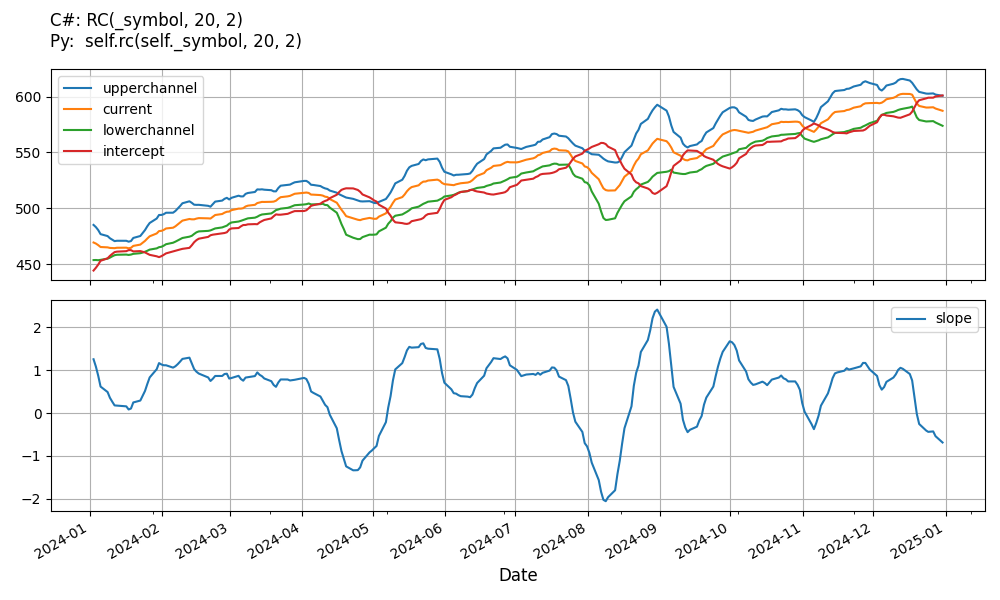# Supported Indicators

## Regression Channel

### Introduction

The Regression Channel indicator extends the with the inclusion of two (upper and lower) channel lines that are distanced from the linear regression line by a user defined number of standard deviations. Reference: http://www.onlinetradingconcepts.com/TechnicalAnalysis/LinRegChannel.html

To view the implementation of this indicator, see the LEAN GitHub repository.

### Using RC Indicator

To create an automatic indicators for RegressionChannel, call the RC helper method from the QCAlgorithm class. The RC method creates a RegressionChannel object, hooks it up for automatic updates, and returns it so you can used it in your algorithm. In most cases, you should call the helper method in the Initialize method.

public class RegressionChannelAlgorithm : QCAlgorithm
{
private Symbol _symbol;
private RegressionChannel _rc;

public override void Initialize()
{
_rc = RC(_symbol, 20, 2);
}

public override void OnData(Slice data)
{
{
// The current value of _rc is represented by itself (_rc)
// or _rc.Current.Value
Plot("RegressionChannel", "rc", _rc);
// Plot all properties of rc
Plot("RegressionChannel", "linearregression", _rc.LinearRegression);
Plot("RegressionChannel", "upperchannel", _rc.UpperChannel);
Plot("RegressionChannel", "lowerchannel", _rc.LowerChannel);
Plot("RegressionChannel", "intercept", _rc.Intercept);
Plot("RegressionChannel", "slope", _rc.Slope);
}
}
}
class RegressionChannelAlgorithm(QCAlgorithm):
def Initialize(self) -> None:
self.rc = self.RC(self.symbol, 20, 2)

def OnData(self, slice: Slice) -> None:
# The current value of self.rc is represented by self.rc.Current.Value
self.Plot("RegressionChannel", "rc", self.rc.Current.Value)
# Plot all attributes of self.rc
self.Plot("RegressionChannel", "linearregression", self.rc.LinearRegression.Current.Value)
self.Plot("RegressionChannel", "upperchannel", self.rc.UpperChannel.Current.Value)
self.Plot("RegressionChannel", "lowerchannel", self.rc.LowerChannel.Current.Value)
self.Plot("RegressionChannel", "intercept", self.rc.Intercept.Current.Value)
self.Plot("RegressionChannel", "slope", self.rc.Slope.Current.Value)


The following reference table describes the RC method:

### RC()1/1

            RegressionChannel QuantConnect.Algorithm.QCAlgorithm.RC (
Symbol                           symbol,
Int32                            period,
Decimal                          k,
*Nullable<Resolution>      resolution,
*Func<IBaseData, Decimal>  selector
)


Creates a new RegressionChannel indicator which will compute the LinearRegression, UpperChannel and LowerChannel lines, the intercept and slope.

If you don't provide a resolution, it defaults to the security resolution. If you provide a resolution, it must be greater than or equal to the resolution of the security. For instance, if you subscribe to hourly data for a security, you should update its indicator with data that spans 1 hour or longer.

You can manually create a RegressionChannel indicator, so it doesn't automatically update. Manual indicators let you update their values with any data you choose.

Updating your indicator manually enables you to control when the indicator is updated and what data you use to update it. To manually update the indicator, call the Update method with time/number pair, or an IndicatorDataPoint. The indicator will only be ready after you prime it with enough data.

public class RegressionChannelAlgorithm : QCAlgorithm
{
private Symbol _symbol;
private RegressionChannel _rc;

public override void Initialize()
{
_rc = new RegressionChannel(20, 2);
}

public override void OnData(Slice data)
{
if (data.Bars.TryGeValue(_symbol, out var bar))
{
_rc.Update(bar.EndTime, bar.Close);
}

{
// The current value of _rc is represented by itself (_rc)
// or _rc.Current.Value
Plot("RegressionChannel", "rc", _rc);
// Plot all properties of rc
Plot("RegressionChannel", "linearregression", _rc.LinearRegression);
Plot("RegressionChannel", "upperchannel", _rc.UpperChannel);
Plot("RegressionChannel", "lowerchannel", _rc.LowerChannel);
Plot("RegressionChannel", "intercept", _rc.Intercept);
Plot("RegressionChannel", "slope", _rc.Slope);
}
}
}
class RegressionChannelAlgorithm(QCAlgorithm):
def Initialize(self) -> None:
self.rc = RegressionChannel(20, 2)

def OnData(self, slice: Slice) -> None:
bar = slice.Bars.get(self.symbol)
if bar:
self.rc.Update(bar.EndTime, bar.Close)

# The current value of self.rc is represented by self.rc.Current.Value
self.Plot("RegressionChannel", "rc", self.rc.Current.Value)
# Plot all attributes of self.rc
self.Plot("RegressionChannel", "linearregression", self.rc.LinearRegression.Current.Value)
self.Plot("RegressionChannel", "upperchannel", self.rc.UpperChannel.Current.Value)
self.Plot("RegressionChannel", "lowerchannel", self.rc.LowerChannel.Current.Value)
self.Plot("RegressionChannel", "intercept", self.rc.Intercept.Current.Value)
self.Plot("RegressionChannel", "slope", self.rc.Slope.Current.Value)


To register a manual indicator for automatic updates with the security data, call the RegisterIndicator method.

public class RegressionChannelAlgorithm : QCAlgorithm
{
private Symbol _symbol;
private RegressionChannel _rc;

public override void Initialize()
{
_rc = new RegressionChannel(20, 2);
RegisterIndicator(_symbol, _rc, Resolution.Daily);
}

public override void OnData(Slice data)
{
{
// The current value of _rc is represented by itself (_rc)
// or _rc.Current.Value
Plot("RegressionChannel", "rc", _rc);
// Plot all properties of rc
Plot("RegressionChannel", "linearregression", _rc.LinearRegression);
Plot("RegressionChannel", "upperchannel", _rc.UpperChannel);
Plot("RegressionChannel", "lowerchannel", _rc.LowerChannel);
Plot("RegressionChannel", "intercept", _rc.Intercept);
Plot("RegressionChannel", "slope", _rc.Slope);
}
}
}
class RegressionChannelAlgorithm(QCAlgorithm):
def Initialize(self) -> None:
self.rc = RegressionChannel(20, 2)
self.RegisterIndicator(self.symbol, self.rc, Resolution.Daily)

def OnData(self, slice: Slice) -> None:
# The current value of self.rc is represented by self.rc.Current.Value
self.Plot("RegressionChannel", "rc", self.rc.Current.Value)
# Plot all attributes of self.rc
self.Plot("RegressionChannel", "linearregression", self.rc.LinearRegression.Current.Value)
self.Plot("RegressionChannel", "upperchannel", self.rc.UpperChannel.Current.Value)
self.Plot("RegressionChannel", "lowerchannel", self.rc.LowerChannel.Current.Value)
self.Plot("RegressionChannel", "intercept", self.rc.Intercept.Current.Value)
self.Plot("RegressionChannel", "slope", self.rc.Slope.Current.Value)


The following reference table describes the RegressionChannel constructor:

### RegressionChannel()1/2

            RegressionChannel QuantConnect.Indicators.RegressionChannel (
string   name,
int      period,
decimal  k
)


Initializes a new instance of the RegressionChannel class.

### RegressionChannel()2/2

            RegressionChannel QuantConnect.Indicators.RegressionChannel (
int      period,
decimal  k
)


Initializes a new instance of the LeastSquaresMovingAverage class.

### Visualization

The following image shows plot values of selected properties of RegressionChannel using the plotly library.You can also see our Videos. You can also get in touch with us via Discord.